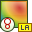# OF Card

This card specifies an offset for the origin of the coordinate system for near and far field calculations. It also facilitates using only a part of the structure through label selection when calculating fields.

On the Request tab, in the Solution requests group, click theRequest options (OF) icon.

## Parameters:

Field calculation for all structures
Near and far fields are calculated for all structures.
Field calculation for structures with specified labels(s)
Use label selection when calculating near and far fields. Only the currents on structures with specified labels are used during field computation.
Field calculation for structures with label range
Use label selection when calculating near and far fields. Only the currents on structures with a label in the range specified in the fields Start at label and End at label are used during field computation. (If a basis function extends over, for example, two triangles it is included if either triangle is in the specified range.)
Calculate near field or far field in local coordinate system
Use the offset specified below as the origin of the coordinate system for field calculations.
Origin of offset coordinate
In this group the Cartesian coordinates of the transformed origin are specified. Each of x, y and z is scaled by the SF card if the SF card is used.
The angle of rotation ${\alpha }_{x}$ around the X axis, the angle of rotation ${\alpha }_{y}$ around the Y axis and the angle of rotation ${\alpha }_{z}$ around the Z axis in degrees.